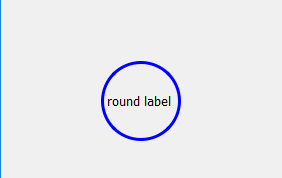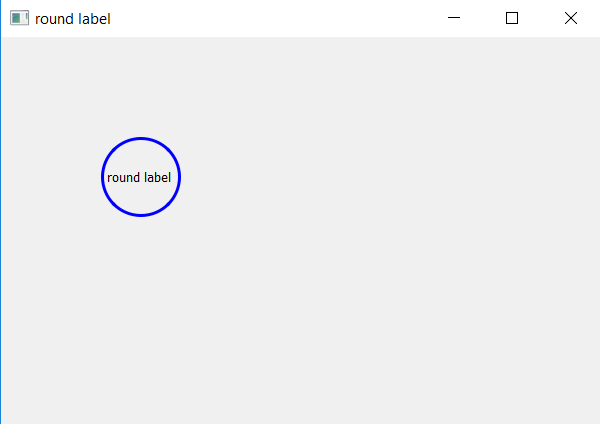# PyQt5 – How to create circle Label ?

• Difficulty Level : Basic
• Last Updated : 26 Mar, 2020

When we create label, by default, they are rectangle in shape, we cane use `resize()` method to change its width and height but still it will be quadrilateral.

In this article, we will see how to create circular i.e round label. In order to do this we will first create a square label then with the help of `setStyleSheet()` method change its border radius to half of length of the label, which will look like this.Argument : It takes string as argument.

Action performed : Changes the border radius of label.

Code :

 `# importing the required libraries`` ` `from` `PyQt5.QtWidgets ``import` `*` `from` `PyQt5.QtGui ``import` `*` `from` `PyQt5 ``import` `QtCore``from` `PyQt5.QtCore ``import` `Qt``import` `sys`` ` ` ` `class` `Window(QMainWindow):``    ``def` `__init__(``self``):``        ``super``().__init__()`` ` ` ` `        ``# set the title``        ``self``.setWindowTitle(``"round label"``)`` ` `        ``# setting  the geometry of window``        ``self``.setGeometry(``60``, ``60``, ``600``, ``400``)`` ` `        ``# creating a label widget``        ``# by default label will display at top left corner``        ``self``.label_1 ``=` `QLabel(``'round label'``, ``self``)`` ` `        ``# moving position``        ``self``.label_1.move(``100``, ``100``)`` ` `        ``# making label square in size``        ``self``.label_1.resize(``80``, ``80``)`` ` `        ``# setting up border and radius``        ``self``.label_1.setStyleSheet("border: ``3px` `solid blue;``                                    ``border``-``radius: ``40px``;")`` ` `        ``# show all the widgets``        ``self``.show()`` ` ` ` `# create pyqt5 app``App ``=` `QApplication(sys.argv)`` ` `# create the instance of our Window``window ``=` `Window()`` ` `# start the app``sys.exit(App.``exec``())`

Output :My Personal Notes arrow_drop_up